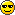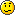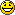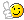# Clients on same subnet?

I was working through the exercises in the MS Press (2nd Edition) book (Chapter 2, Lesson 3, Lesson Review #4), and struggled a bit to determine the best way to figure out how two clients are on the same subnet. Here's the question;

You have obtained the 192.168.0.0/20 address block from a central network administrator. Within your network, you want the addresses 192.168.3.1 and 192.168.4.1 to belong to the same subnet, but you also want to maximize the number of subnets available. Which subnet mask should you configure within your organization?

A) /20/21
C) /22
D) /23

According to the MS Press book, in order to figure out if two clients on the same subnet, use this formula;

k1 = value of octect in IP address 1
k2 = value of octect in IP address 2

k1 / (256-d) = ___
k2 / (256-d) = ___

If those two numbers are the same, then they are on the same subnet. Easy enough, until I try to use the values for the answers to figure it out;

A) /20 = 255.255.240.0
3 / (256-240) = 3 / 16 = 0.1875
4 / (256-240) = 4 / 16 = 0.25
--- Different numbers, Not on same subnet/21 = 255.255.248.0
3 / (256-24= 3 / 8 = 0.375
4 / (256-24= 4 / 8 = 0.5
--- Different numbers, Not on same subnet

C) /22 = 255.255.252.0
3 / (256-252) = 3 / 4 = 0.75
4 / (256-252) = 4 / 4 = 1
--- Different numbers, Not on same subnet

D) /23 = 255.255.254.0
3 / (256-254) = 3 / 2 = 1.5
4 / (256-254) = 4 / 2 = 2
--- Different numbers, Not on same subnet

MS Press seems to think the correct answer is B (/21) -- I'm unable to produce this using their suggested formula.Anyone have a better way to figure this out? Or is able to explain this more clearly?

Thanks!

• Let's see...

/20 would give you a 255.255.240.0 subnet mask. So you would have subnets such as 192.168.0.0, 192.168.16.0, 192.168.32.0, etc. ( 256 - 240 = 16 so your subnets increment by 16 )

/21 would give you a 255.255.248.0 subnet mask. So you would have subnets such as 192.168.0.0, 192.168.8.0, 192.168.16.0, etc. ( 256 - 248 = 8 so your subnets increment by 8 )

/22 would give you a 255.255.252.0 subnet mask. So you would have subnets such as 192.168.0.0, 192.168.4.0, 192.168.8.0, etc. ( 256 - 252 = 4 so your subnets increment by 4 ). Notice that 192.168.4.1 would be on a different subnet, so this is not the answer.

/23 would give you a 255.255.254.0 subnet mask. So you would have subnets such as 192.168.0.0, 192.168.2.0, 192.168.4.0, etc. ( 256 - 254 = 2 so your subnets increment by 2 ). Notice that 192.168.4.1 would be on a different subnet, so this is not the answer.

Since you want to maximize the number of subnets, you'd have to use /21 as it provides for more subnets because you're incrementing them by 8, not 16 and the first requirement is also met as both hosts, 192.168.3.1 and 192.168.4.1 would be on the same subnet.

Hope this helps,

IP
Every day hurts, the last one kills.
• IP - thanks for the reply and explanationI knew that, but it seems to stick now that I see someone else writing itAny ideas why the book would suggest you divide the IP octet (in this example 192.168.3.0 and 192.168.4.0) to get a number to compare? I would think it would be easier to just see if the numbers fit in the increments...
• Member Posts: 30 ■■□□□□□□□□
Hi,

I was going to ask a similar question to jdotq, but this thread answered most of itI have a query that I hope someone can help.

to qoute part of ipchains answer.
/20 would give you a 255.255.240.0 subnet mask. So you would have subnets such as 192.168.0.0, 192.168.16.0, 192.168.32.0, etc. ( 256 - 240 = 16 so your subnets increment by 16 )

From the subnet mask of 255.255.240.0, what is the best way to determine the amount of hosts each subnet can support and the maximum subnets supported?

My study material advises number of hosts = 2(power of x) where x is number of host bits used for subnet id.
is this correct?
It then says that the number of hosts is determined by the following formula (2x)-2. Where x is remaining bits left for host id.
It seems the book has given me 2 different formulas for the same thing, so I'm a it confused. It's the exam cram series book.

Thank You
Do just once what others say you can't do, and you will never pay attention to their limitations again.
• exit12,

To find hosts on a network (for this example 255.255.240.0);

For the network, you have two segments of bits, the network bits and the host bits -- in the example the network bits is 20 and the host bits is 12 (32 total minus 20 used for the network)

The hosts 'formula' is (2^x)-2, where "x" is the host bits. So, in the example the formula would be (2^12)-2 = (4096)-2 = 4094.

You subtract a value of 2 from 4096 due to two addresses being reserved for the network ID and the broadcast address. So the total number of available hosts would be 4094

For the maximum amount of subnets, you would use the same formula, but there is no need to subtract 2 for the reserved host addresses; so the formula is 2^x, where "x" is the subnet bits.

But, there are only subnet bits present if you custom subnet! If it's just a default subnet of something like /8, /16, /24 - then the subnet is just 1 -- as soon as you extend the network bits is when you custom subnet;

/20 in binary (the default network given in the example);
11111111.11111111.11110000.00000000

/21 in binary (the answer given in the example);
11111111.11111111.11111000.00000000

Notice the 'new' bits are now the subnet bits! Although it's just one bit in this example, it's now the subnet bit. Bits before the subnet are the network bits, and bits after the subnet are the host bits;

Network bits;
11111111.11111111.1111____.________

Subnet bits;
________.________.____1___.________

Host bits;
________.________._____000.00000000

So, back to the formula (2^x), where "x" is the subnet bits -- (2^1) = 2.

So maximum subnets is 2, maximum hosts per subnet is 4094

Hope this makes sense!
• Member Posts: 30 ■■□□□□□□□□
Now I'm even more confused!Just the last part thats got me.

I thought a subnet mask of /21 would give 32 subnets? 2^5=32?

I ned to read some more I thinkGood answer though and some things are more clearer!
Do just once what others say you can't do, and you will never pay attention to their limitations again.
• Member Posts: 3,352 ■■■■□□□□□□
Looking good JdotQ. Looks like you're going to do well on the 70-291 subnetting portion.

exit12 wrote:
I thought a subnet mask of /21 would give 32 subnets? 2^5=32?

You are correct, but that is what JdotQ said:
/21 = 1111111.11111111.11111000.0000000

5 1s in the subnet portion = 2^5 = 32

Nowhere did JdotQ say that /21 was not 32 subnets.
“For success, attitude is equally as important as ability.” - Harry F. Banks
• royal wrote:
Looking good JdotQ. Looks like you're going to do well on the 70-291 subnetting portion.
Thanks royalMaybe it would've helped in my explanation if I mentioned the IP is a class B (192.168.x.x), so default subnet would be /16 (255.255.0.0). Anything for the subnet greater than the default, would be the subnet bits...

So for /21;

Network Bits (default for Class:
11111111.11111111.11111000.0000000

Subnet Bits (extended from the default):
11111111.11111111.11111000.00000000

Host Bits (remaining bits after subnet bits):
11111111.11111111.11111000.00000000
• Member Posts: 30 ■■□□□□□□□□This thread has made subnetting a whole lot clearer and easier to understand than it was from my study books!

Big thanks Jdotq!Do just once what others say you can't do, and you will never pay attention to their limitations again.
• exit12 wrote:This thread has made subnetting a whole lot clearer and easier to understand than it was from my study books!

Big thanks Jdotq!Glad to hear it finally 'clicked'!The MS Press book does an ok job of presenting subnetting -- but I think CBT Nuggets does a MUCH better job, especially with the parts on custom subnetting; if you have access to CBT Nuggets, I would highly recommend them for this exam.